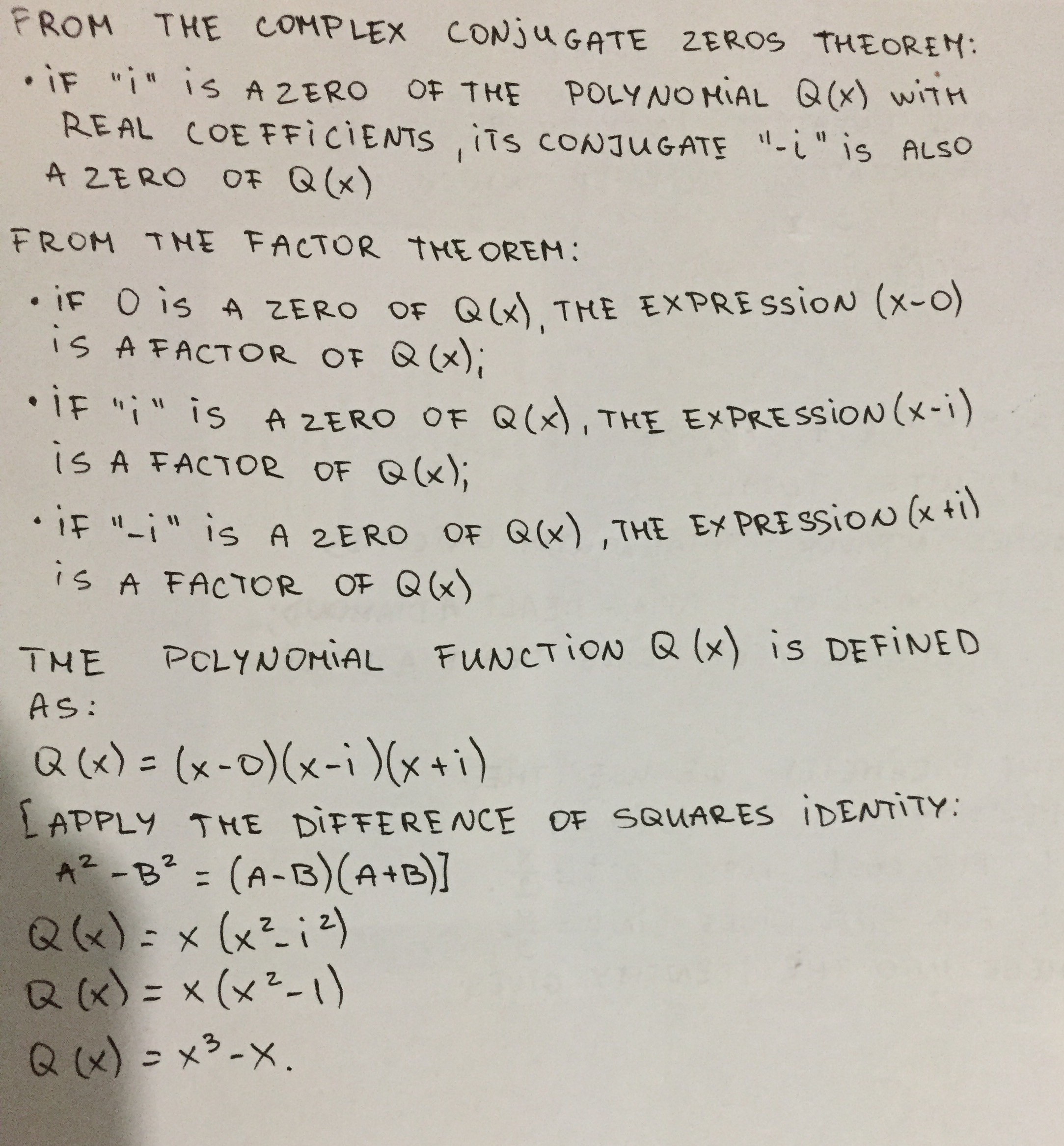Albarellak

2020-10-28

Find a polynomial with integer coefficients that satisfies the given conditions. Q has degree 3 and zeros 0 and i.Liyana Mansell

From the Complex Conjugate Zeros Theorem:
If “i” is a zero of the polynomial Q(x) with real coefficients, its conjugate “-i” is also a zero of Q (x)
From the factor theorem:
If 0 is a zero of Q (x) the expression (x – 0) is a factor of Q (x).
If “i” is a zero of Q (x) the expression (x – i) is a factor of Q (x).
If “-i” is a zero of Q (x) the expression (x + i) is a factor of Q (x).
The polynomial function Q (x) is defined as:
$Q\left(x\right)=\left(x–0\right)\left(x–i\right)\left(x+i\right)$
[apply the difference of squares identity: ${a}^{2}–{b}^{2}=\left(a-b\right)\left(a+b\right)\right]$
$Q\left(x\right)=x\left({x}^{2}–{i}^{2}\right)$
$Q\left(x\right)=x\left({x}^{2}+1\right)$
$Q\left(x\right)={x}^{3}+x$2021-07-16

Q(x)=x^2(x-i)(x+i)

Do you have a similar question?